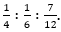Dear Aspirants,

Quantitative Aptitude Quiz For SBI PO/Clerk Prelims

Numerical Ability or Quantitative Aptitude Section has given heebie-jeebies to the aspirants when they appear for a banking examination. As the level of every other section is only getting complex and convoluted, there is no doubt that this section, too, makes your blood run cold. The questions asked in this section are calculative and very time-consuming. But once dealt with proper strategy, speed, and accuracy, this section can get you the maximum marks in the examination. Following is the Quantitative Aptitude quiz to help you practice with the best of latest pattern questions.

Q1. A and B entered into a partnership, investing Rs. 16000 and Rs. 12000 respectively. After 3 months, ‘A’ withdraws Rs. 5000, while B invested Rs. 5000 more. After 3 months more, C joins the business with a capital of Rs. 21,000. After an year, they obtained a profit of Rs. 26400. What is B’s share in the profit?
Rs.10050
Rs.11600
Rs.10500
Rs.10800
None of these

Q2. A is an active partner and B is an inactive partner in business. A put in Rs. 5,000 and B puts in Rs. 6,000. A received 15% of the total profit for managing the business and the rest is divided in ratio of their invested capitals. Then find the amount received by A out of the total profit of Rs. 880 ?
Rs. 132
Rs. 340
Rs. 472
Rs. 492
Rs. 452

Q3. A, B and C become partners in a business. A contributes ⅓ rd of the capital for ¼ th of the time. B contributes ⅕ th of the capital for 1/6 th of the time and C the rest of the capital for the whole time. If the profit is Rs. 1,820, then find the share of A out of total profit?
Rs. 130
Rs. 260
Rs. 292
Rs. 304
Rs. 312

Q4. A, B and C share profit in the ratio ofIf C retires, A and B share the profit of C in the ratio of 4 : 5 respectively. Then what will be the new profit sharing ratio of A and B ?
55 : 53
53 : 55
5 : 3
3 : 5
3 : 7

Q5. A and B enter into partnership, investing Rs. 48,000 and Rs. 60,000 respectively. After 3 months, A withdraws Rs. 8,000 while B invests Rs. 6,000 after 6 months of starting of business. Out of the total amount of profit, if A gets Rs. 12,000 as his share at the end of the year,then find total profit share of A and B together?
Rs. 24,000
Rs. 30,000
Rs. 36,000
Rs. 37,000
Rs. 38,000

Q6. M, P and Q together started a business. M invested Rs. 6,500 for 6 months, P invested Rs. 8,400 for 5 months, and Q invested Rs. 10,000 for 3 months. M is working member for which he gets 5% of total profit extra and rest profit divided in ratio of their invested capitals. If the total profit is Rs. 7400, then find Q’s share?
Rs. 1900
Rs. 2,100
Rs. 3,200
Data are incomplete
Rs. 3,600

Q7. A starts a business with an initial investment of Rs 18000. After 4 months, B enters into the partnership with an investment of Rs 24000. Again after two months, C enters with an investment of Rs 30000. If C receives Rs 1845 in the profit at the end of the year, what is total annual profit?
Rs 6027
Rs 6327
Rs 6527
Rs 6080
Rs 6800

Q8. A, B and C started a business by investing Rs 20,000, Rs 28,000 and Rs 36,000 respectively. After 6 months, A and B withdrew an amount of Rs 8,000 each and C invested an additional amount of Rs 8,000. All of them invested for equal period of time. If at the end of the year, C got Rs 12,550 as his share of profit, what was the total profit earned?
Rs 25,100
Rs 26,600
Rs 24,300
Rs 22,960
Rs 21,440

Q9. A discount of 15% is given on the marked price of an article. The shopkeeper charges sales tax of 6% on the discounted price. If the selling price be Rs 1081.20, what is the marked price of the article?
Rs 1185.20
Rs 1250.20
Rs 302
Rs 1200
Rs 1205.50

Q10. A sells goods to B at five-thirds to that rate of profit at which B has decided to sell it to C. C, on the other hand, sells it to D at one-third the rate of profit at which B sold it to C. If D gives Rs. 2145 to C at 10% profit, how much did A buy it for?
Rs. 1000
Rs. 2000
Rs. 1500
Rs. 1800
Rs. 1600

Q11. A merchant makes a profit of 20% by selling an article. What would the percentage change have been in the profit percentage had he paid 10% less for it and the customer paid 10% more for it with respect to previous selling price?
120%
125%
133.33%
150%
11.111%

Directions (12-15): What will come in place of (?) in the following number series ?

Q12. 148, 152, 161, 177, ? , 238
208
214
202
198
192

Q13. 339, 355, 323, 371, 307, ?
407
354
328
387
388

Q14. 5, 14, 40, 117, 347, ?
920
745
1124
1036
694

Q15. 12, 24, 96, 576, ? , 46080
3542
3890
4248
4608
3246

You May also like to Read: